Free Printable Worksheets for First Grade

## Learn About Isograms – Word Building ActivityAn Isogram, otherwise known as a “nonpattern word”, is just a fancy way of referring to a word or phrase that has no repeating letters. This particular activity not only introduces the child to Iso...

## Build Your Own Math Problems – Fun Paper Cut OutsThis is a great rainy day activity sheet. This math learning project is a little more interactive in that the child gets to cut out the squares necessary to build the problems and then can either a...

## 3 digit addition worksheet with regrouping (Set 4)Free math addition worksheet with 3 digit numbers. All problems in this set are on addition with regrouping....

## 3 digit addition worksheet with regrouping (Set 3)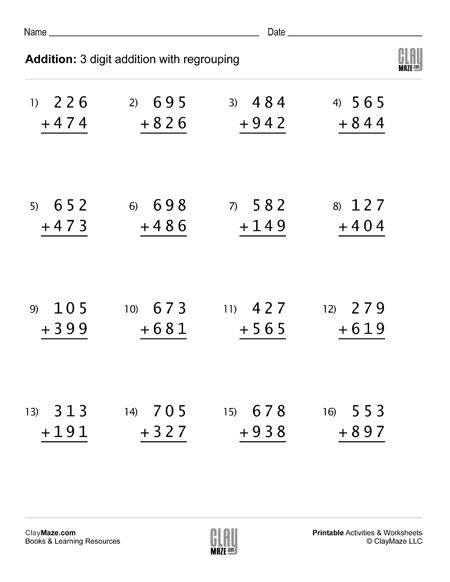This math worksheet contains 16 problems of 3 digit addition with regrouping – good for first grade, second and third grade addition practice....

## 3 digit addition worksheet with regrouping (Set 2)## 3 digit addition worksheet with regrouping (Set 1)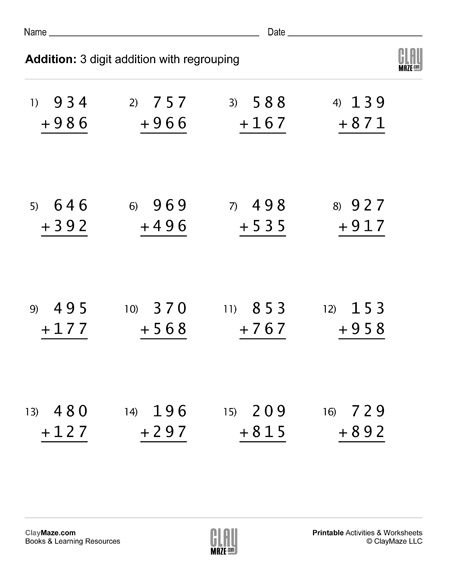## Even Numbers and Odd Numbers (Set 4)Identify the even numbers and odd numbers in this worksheet. Find the even numbers in the first section and then find the odd numbers in the second section. Great for first grade and second grade....

## Even Numbers and Odd Numbers (Set 3)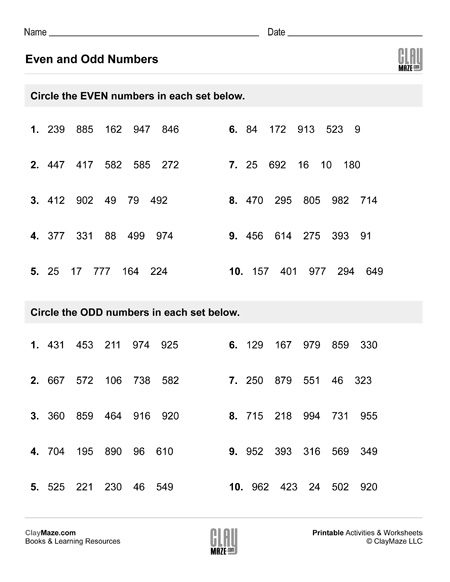Use this worksheet for practice with even numbers and odd numbers. Find the even numbers in each set of the first section, then find the odd numbers in each set of the second section....

## Even Numbers and Odd Numbers (Set 2)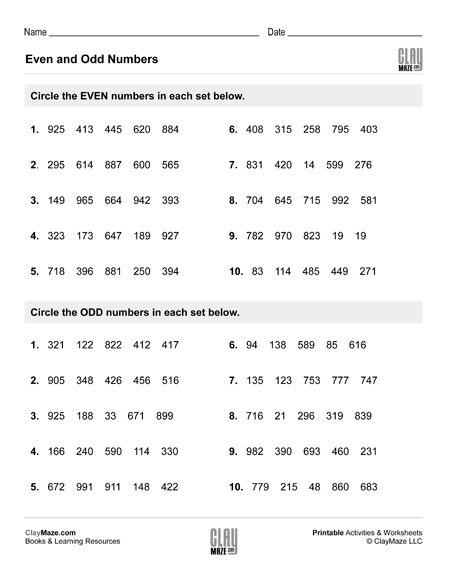Even and odd numbers worksheet. In the first section, the student is asked to circle the even numbers in each group. Then in the second section the student is asked to circle the odd numbers in...

## Even Numbers and Odd Numbers (Set 1)This worksheet is for practice identifying even numbers and odd numbers. It is divided into 2 sections. The student is asked to circle the even numbers in each group in the first section and then...

## Skip Counting Worksheet – Counting by 2’s and Counting by 5’s (Set 4)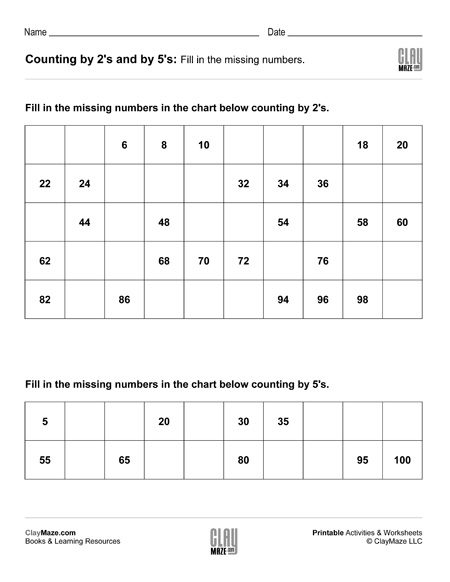Skip counting worksheet – Numbers up to 100. Fill in the missing numbers counting by 2’s and counting by 5’s....

## Skip Counting Worksheet – Counting by 2’s and Counting by 5’s (Set 3)Skip counting worksheet with 2 charts. Counting by 5’s and counting by 2’s....